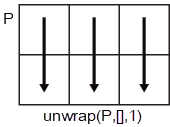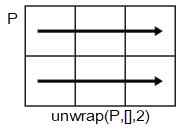# unwrap

## 语法

``Q = unwrap(P)``
``Q = unwrap(P,tol)``
``Q = unwrap(P,[],dim)``
``Q = unwrap(P,tol,dim)``

## 说明

``Q = unwrap(P)` 展开向量 `P` 中的弧度相位角。每当连续相位角之间的跳跃大于或等于 π 弧度时，`unwrap` 就会通过增加 ±2π 的整数倍来平移相位角，直到跳跃小于 π。如果 `P` 是矩阵，`unwrap` 将按列运算。如果 `P` 是多维数组，`unwrap` 将对大小大于 1 的第一个维度进行运算。`

``Q = unwrap(P,tol)` 将 `P` 元素之间的跳跃与跳跃阈值 `tol` 进行比较，而不是与默认值 π 弧度进行比较。如果您指定的跳跃阈值小于 π，unwrap 将使用默认的跳跃阈值 π。`

``Q = unwrap(P,[],dim)` 沿维度 `dim` 展开。`
``Q = unwrap(P,tol,dim)` 使用跳跃阈值 `tol` 沿维度 `dim` 展开。`

## 示例

```t = linspace(0,6*pi,201); x = t/pi.*cos(t); y = t/pi.*sin(t); plot(x,y)``````P = atan2(y,x); plot(t,P)``````Q = unwrap(P); plot(t,Q)``````clear; close all; W = [0:0.4:3, 3.4:0.4:5, 5.4:0.4:7]; P = [-1.5723 -1.5747 -1.5790 -1.5852 -1.5922 -1.6044 -1.6269 -1.6998 1.7252 1.5989 1.5916 1.5708 1.5582 -4.7838 -4.8143 -4.8456 -4.8764 -4.9002]; plot(W,P,'bo-')````plot(W,unwrap(P),'ro-')``plot(W,unwrap(P,5),'ro-')``P = [0 7.07; 0.19 0.98; 6.67 1.18; 0.59 1.37; 0.78 1.56]`
```P = 5×2 0 7.0700 0.1900 0.9800 6.6700 1.1800 0.5900 1.3700 0.7800 1.5600 ```

```dim = 1; P1 = unwrap(P,[],dim)```
```P1 = 5×2 0 7.0700 0.1900 7.2632 0.3868 7.4632 0.5900 7.6532 0.7800 7.8432 ```

```dim = 2; P2 = unwrap(P1,[],dim)```
```P2 = 5×2 0 0.7868 0.1900 0.9800 0.3868 1.1800 0.5900 1.3700 0.7800 1.5600 ```

## 输入参数

• `unwrap(P,[],1)` 沿 `P` 的各列进行运算，并返回每列平移后的相位角。• `unwrap(P,[],2)` 沿 `P` 的各行进行运算，并返回每行平移后的相位角。